|

# 陶博会上新|第36届佛山陶博会超全攻略来了!(附展商名单)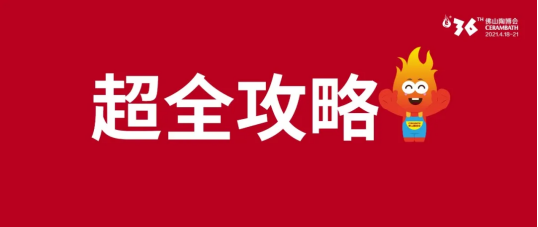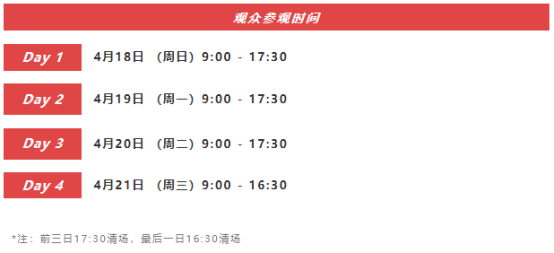Part011、所有进馆人员在参展前做好自我健康状况监测，确保：

①本人及14天内接触人员体温<37.3℃，无咳嗽、腹泻、乏力等症状。

②本人及相关接触人员前14天内无疫情中、高风险旅居史；未被判定为新冠确诊病例、疑似病例、密切接触者或次密接触者。

③完成个人健康申报，提前注册生成广东粤康码绿码，后续现场出示绿码进场。

2、所有进馆人员须全程正确佩戴口罩，有序排队入场，配合体温检测及安全检查，不拥挤，少聚集。

3、本届展会实行全员实名制管理，所有进入展馆人员须在开展前完成预登记注册，凭预登记二维码进入展馆。Part

02👇 展区概况 👇

Part 1▲中国陶瓷城展馆▲A区▲国际精品区1F2F3F4F5F

Part 2▲中国陶瓷总部展馆▲中国陶瓷总部展馆导览图▲岩板家居馆

Part 3▲佛山国际会议展览中心展馆▲佛山国际会议展览中心展馆平面图

👇9大“展中展”主题展区 👇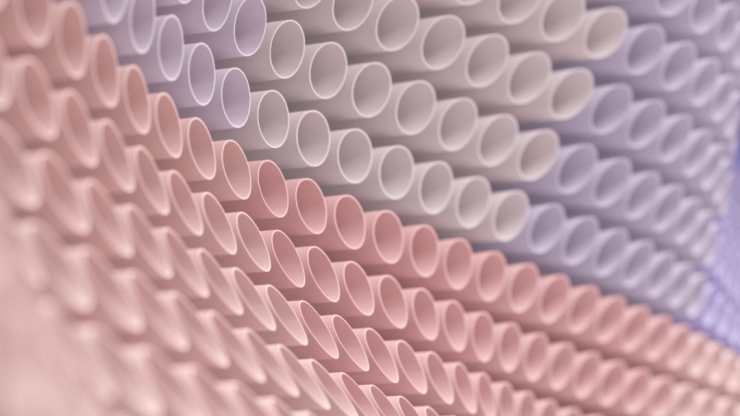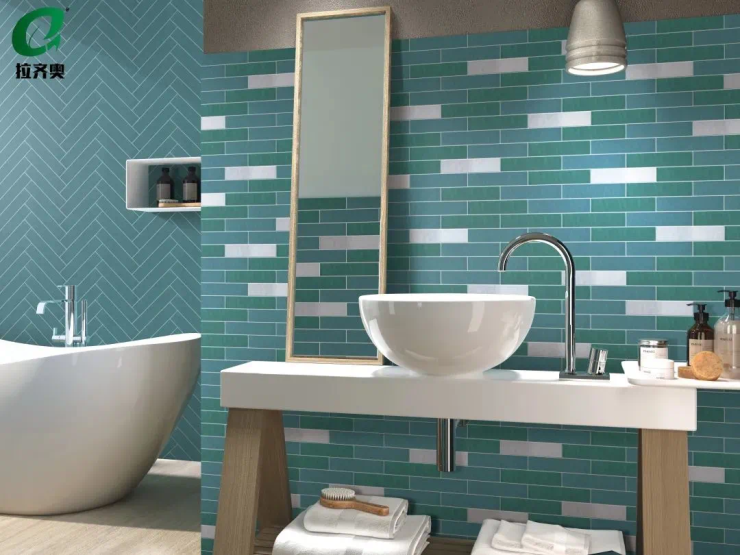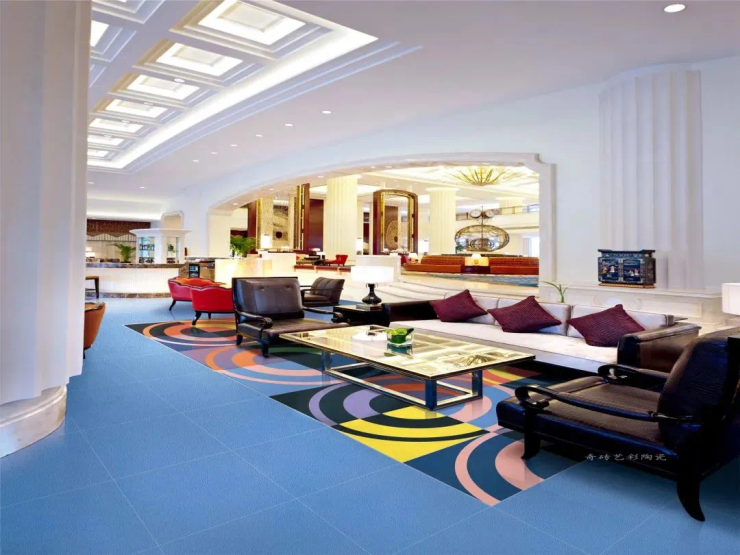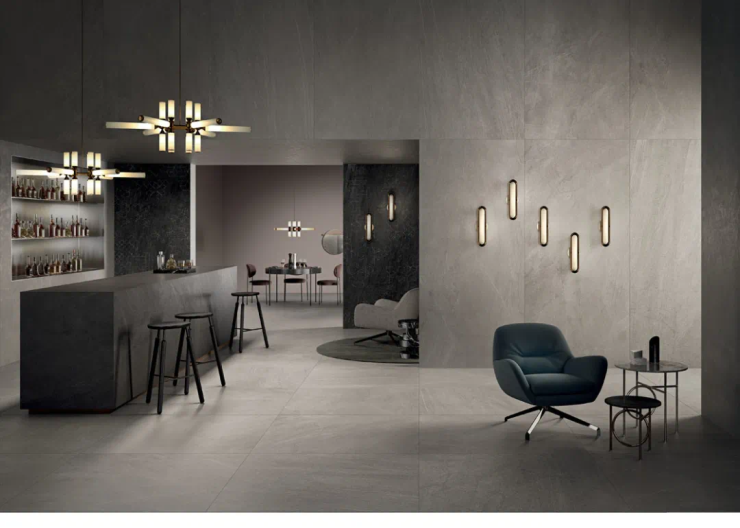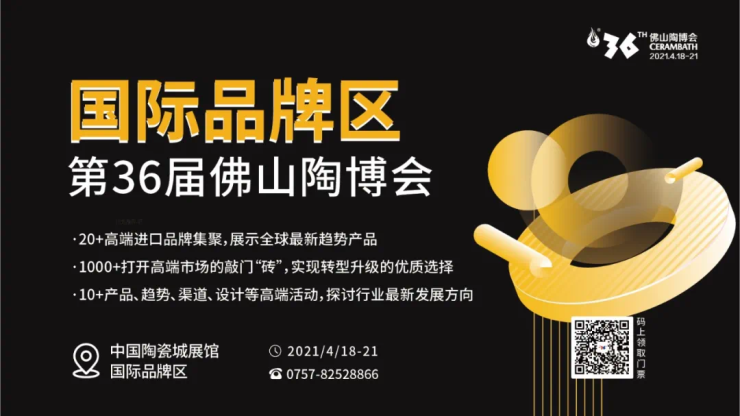“新生艺术节”主题展区

“新生艺术节”主题展区位于中国陶瓷城展馆，由第36届佛山陶博会 X 艺术家刘德志共同推出，刘德志发起策展，用建筑垃圾构建一个艺术装置，并融入茶馆、美术馆等生活业态，同时金意陶、慕瓷、笙花、骏安博、1920等多家品牌强势加入，联袂重现一个关于重生的故事。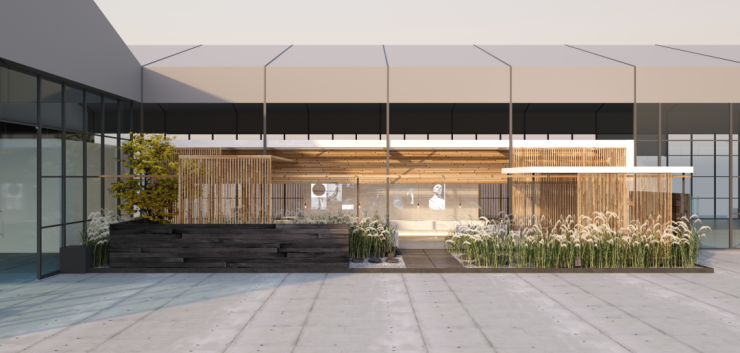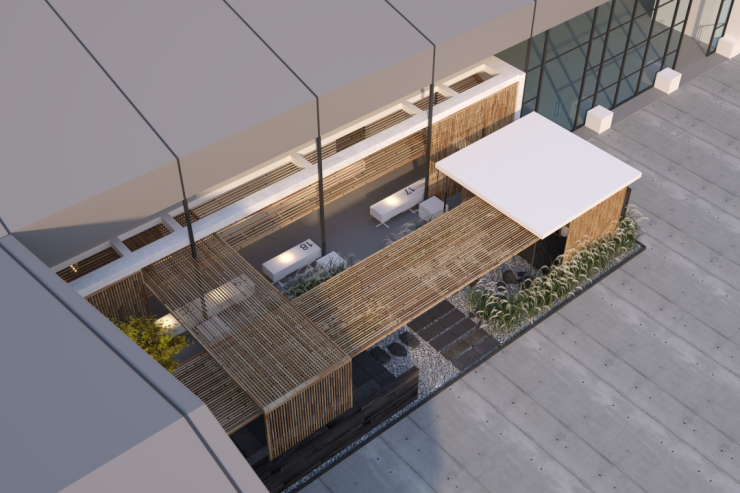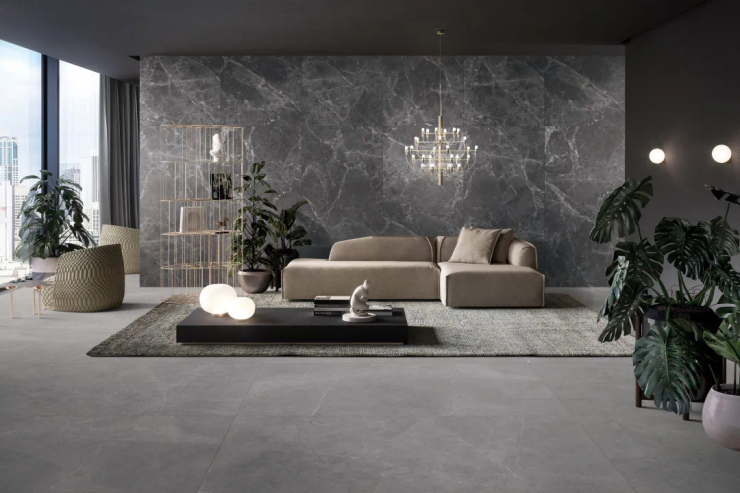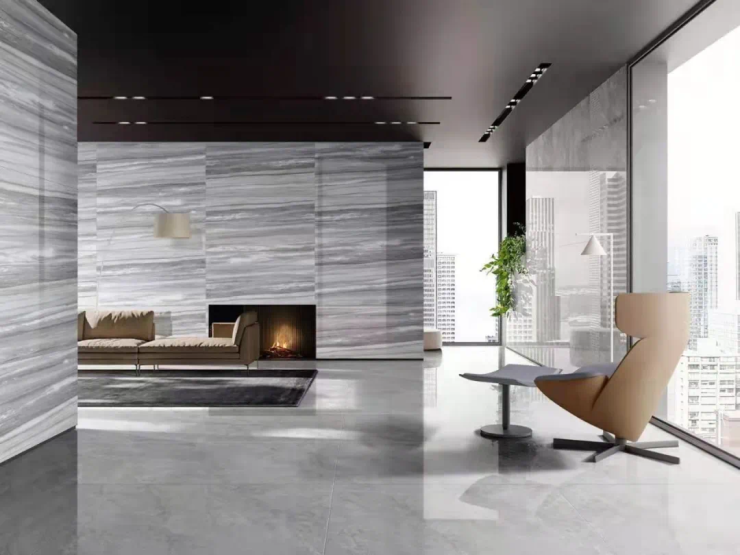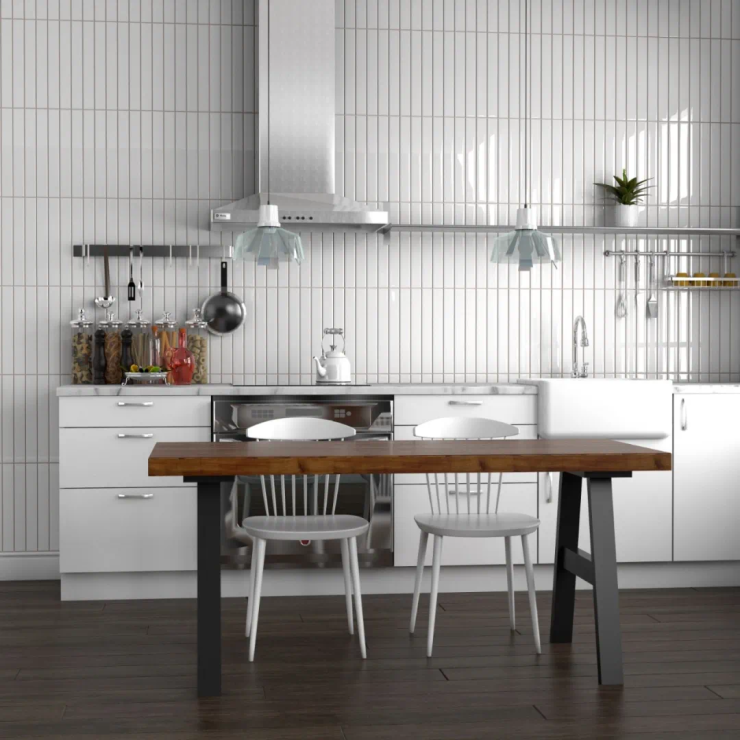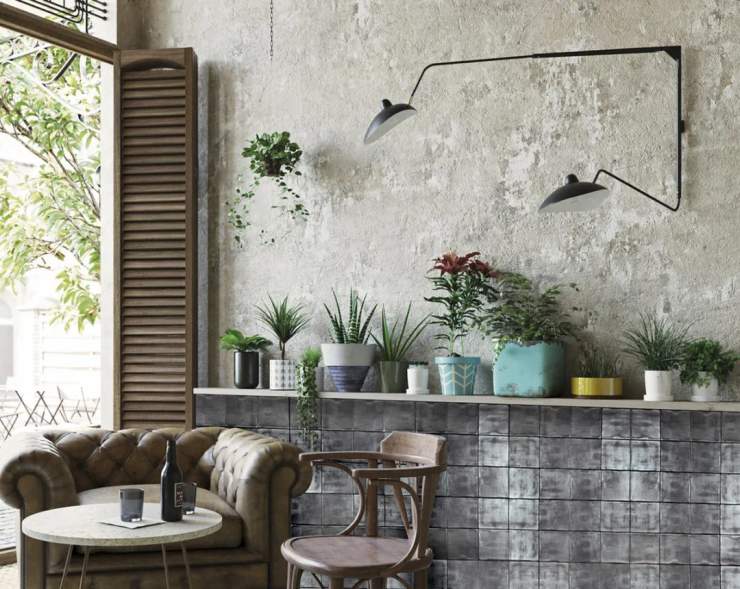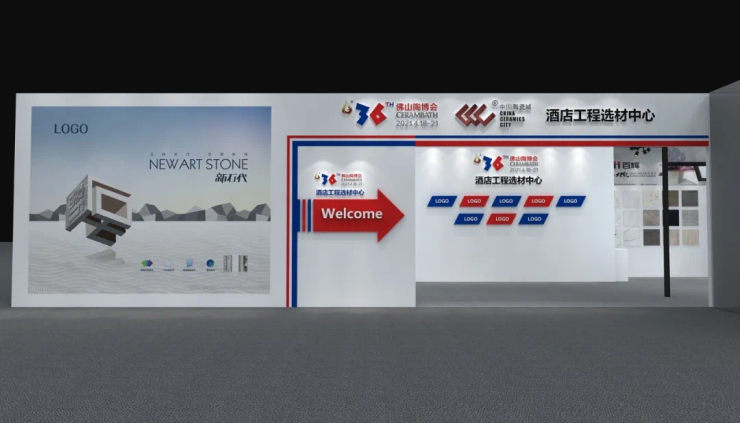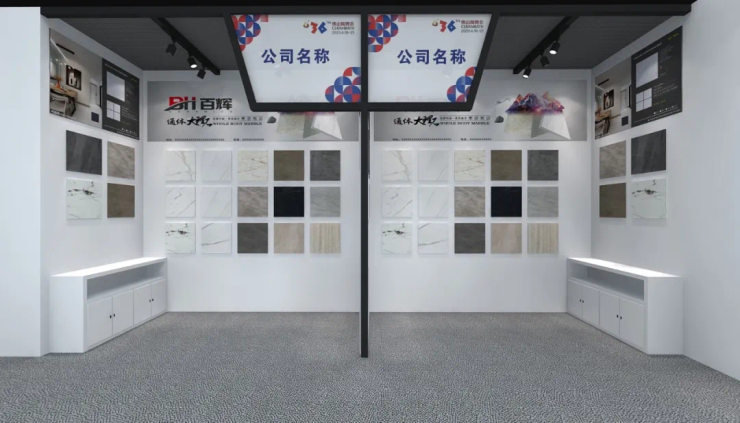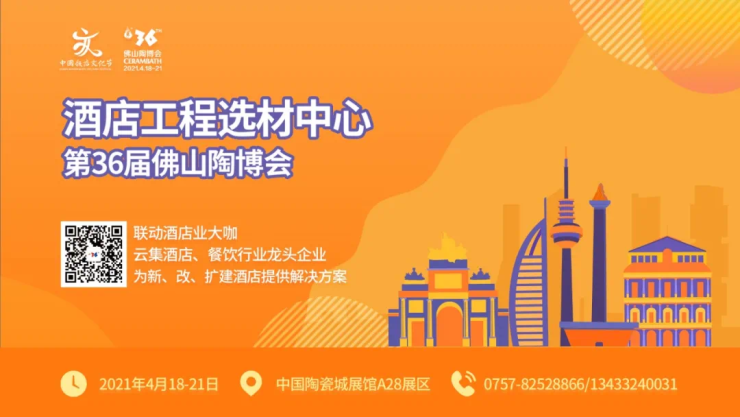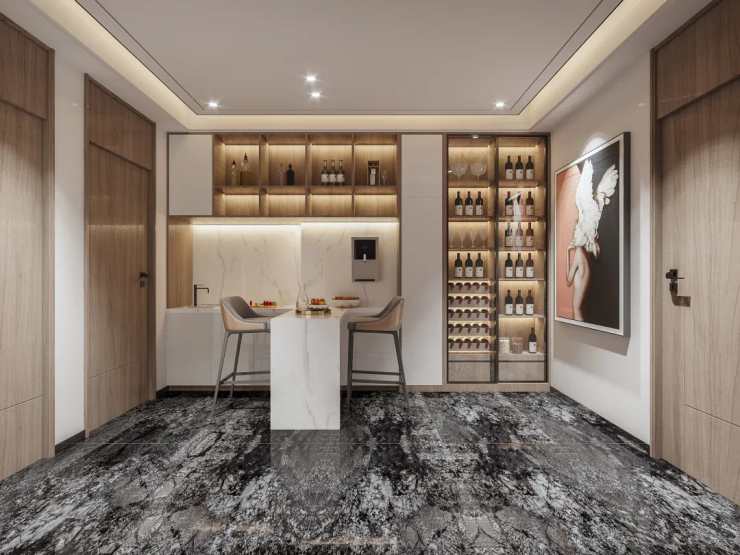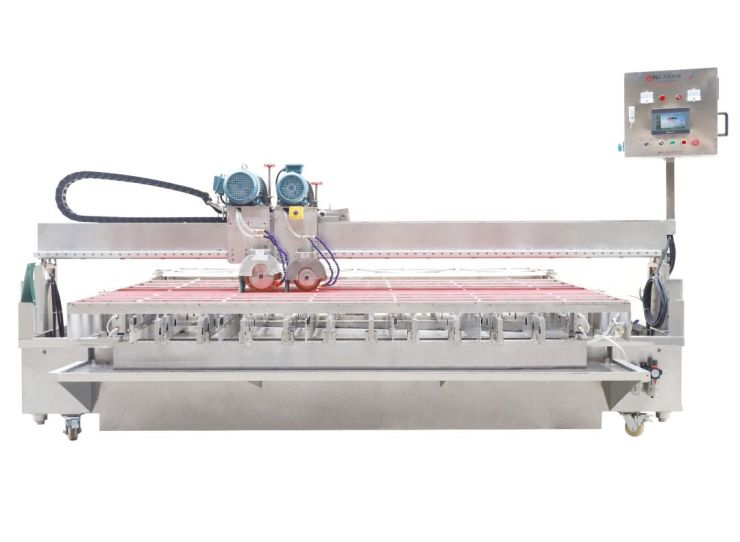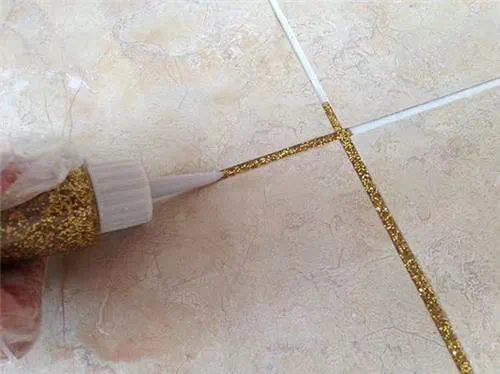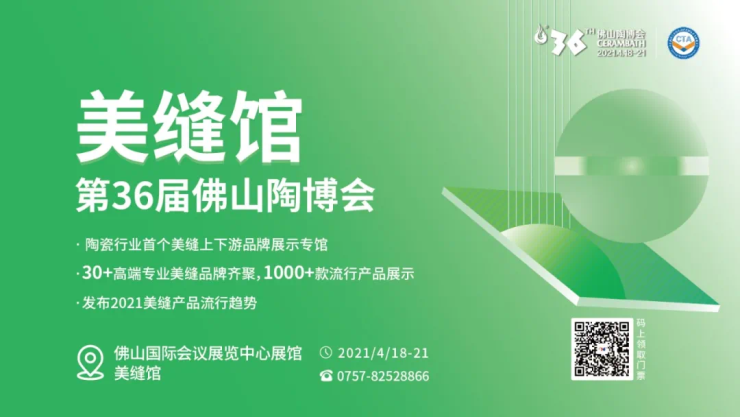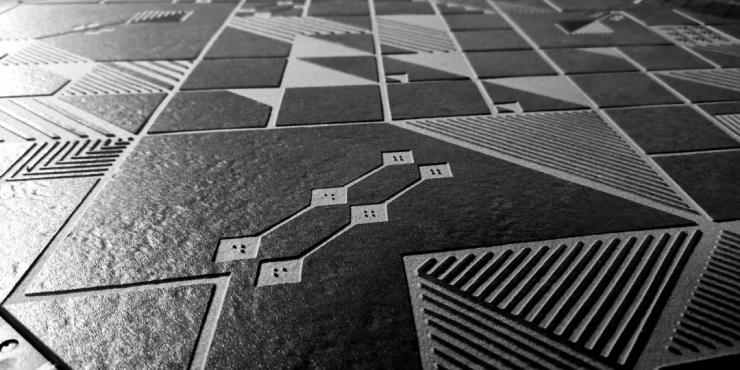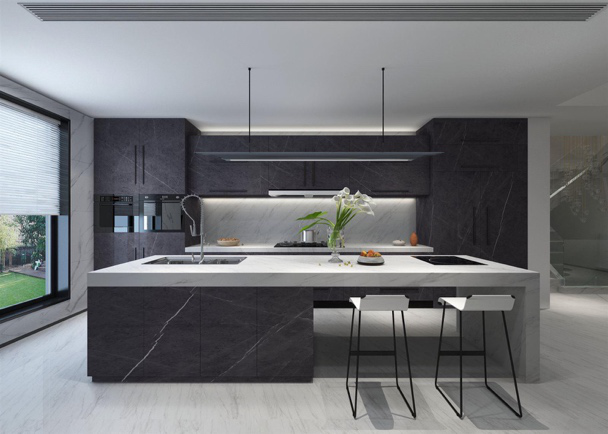👇展期活动 👇

1、第二届佛山陶博会超级直播节2、谋势而动 趋向未来-第七届经销商大会《中国建筑卫生陶瓷行业发展白皮书（2020-2021）》发布

4月19日下午，由中国建筑卫生陶瓷协会、中国建筑材料流通协会、佛山陶瓷行业协会、佛山市陶瓷学会、佛山陶博会组委会主办，《陶业要闻摘要》支持，佛山中国陶瓷城集团有限公司承办的“谋势而动 趋向未来-第七届经销商大会《中国建筑卫生陶瓷行业发展白皮书（2020-2021）》发布将在佛山国际会议展览中心展馆活动A区举行。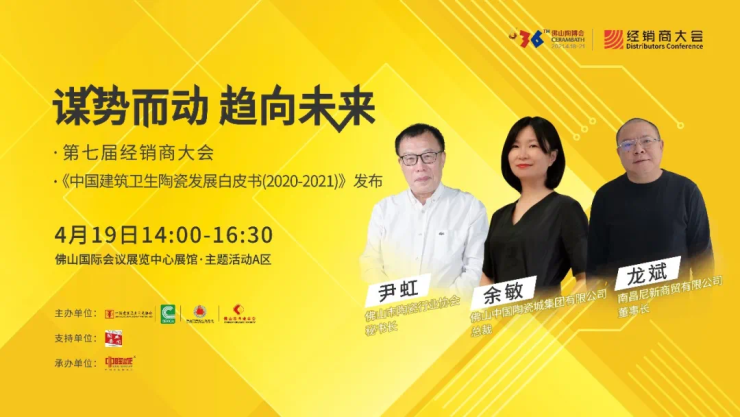3、第二届中国空间设计+创想峰会

4月19日下午，由佛山陶博会与和智有信联合策划的“第二届中国空间设计+创想峰会”在中国陶瓷城展馆主题活动区举行。4、第十届中国饭店文化节暨第五届中国租赁式公寓企业家峰会

4月18日，“第五届中国租赁式公寓企业家峰会”将在第36届佛山陶博会中国陶瓷城展馆活动区举办。本届佛山陶博会链接资源，跨界邀请众多大咖及公寓企业家代表共同探讨，针对宏观政策的演变对住房租赁行业发展的影响，探索中国住房租赁行业公募RELTs发展之路，重磅解读《住房租赁信息指数报告》。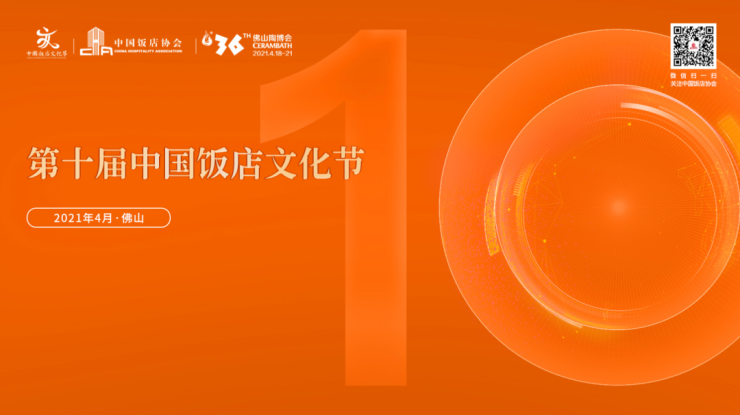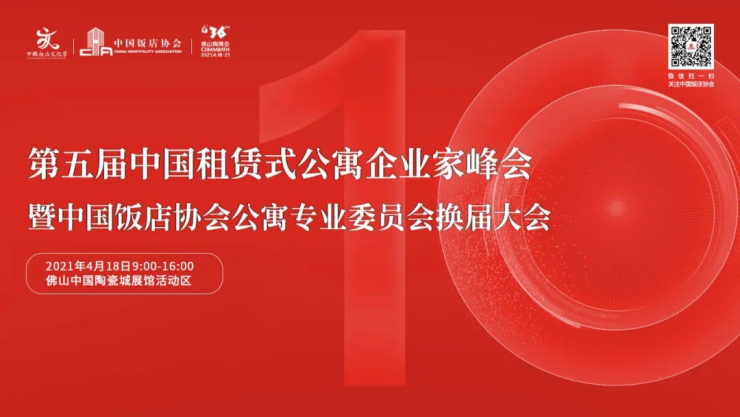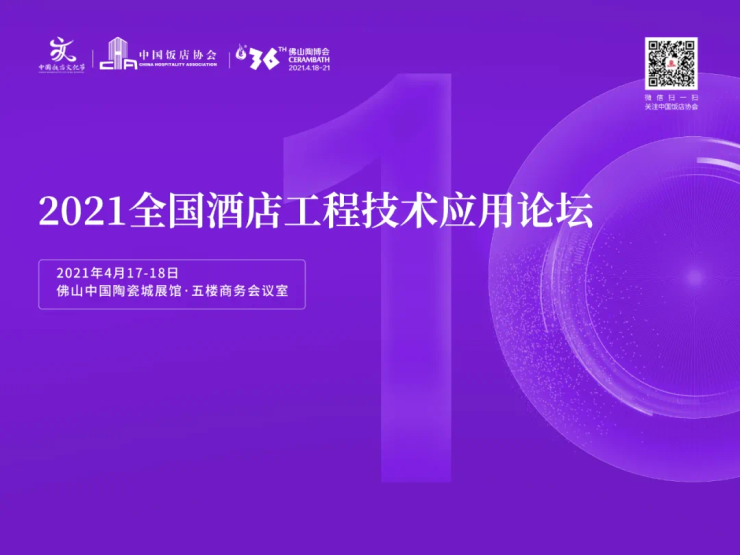5、岩板未来-第36届佛山陶博会经销商岩板考察对接团

4月18日，中陶城、佛山陶博会联合中国岩板·新材展示采购基地即将组织开展经销商岩板考察对接团活动，来自全国各地家具厂、经销商约15人将重点对接华岩品致、LA’BOBO等岩板企业，以达成招商加盟意向。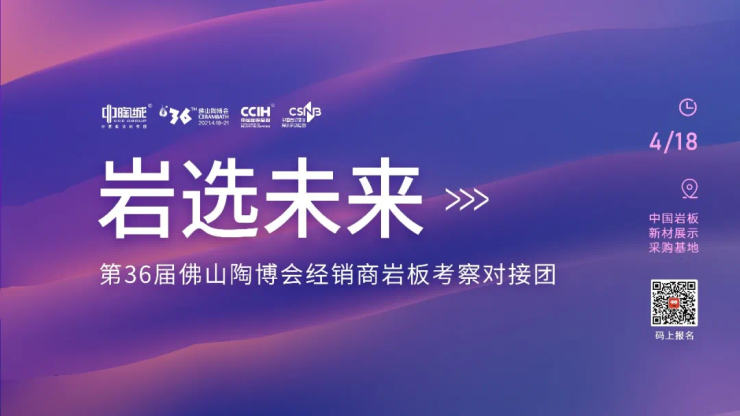6、卫厨高定新浪潮直播招商大会

4月16日晚上7点，中国陶瓷城将联合西普曼高端不锈钢全屋定制、美心淋浴房和MINI定制共同开启“卫厨高定新浪潮”直播招商大会。4月18日，“卫厨高定新浪潮”直播招商大会线下对接团将组织20名经销商重点考察对接直播招商大会意向品牌。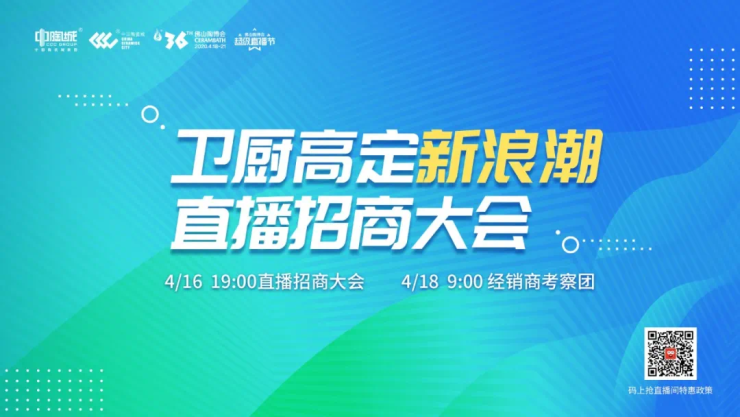7、重新定义建筑岩板-中国岩板应用高峰论坛暨美尔奇品牌战略发布会

4月18日，由中国建筑标准设计研究院、蒙娜丽莎集团、广东绿屋建筑科技工程有限公司、佛山陶博会组委会联合举办“中国岩板应用高峰论坛暨美尔奇品牌战略发布会”。本次活动以“重新定义建筑岩板”为主题，将邀请行业大咖共同探讨岩板在建筑装饰领域的创新应用和发展机遇。同时，活动还将正式发布蒙娜丽莎集团高端岩板品牌—美尔奇的全新品牌形象，全方位展示美尔奇岩板针对建筑领域推出的产品和应用场景。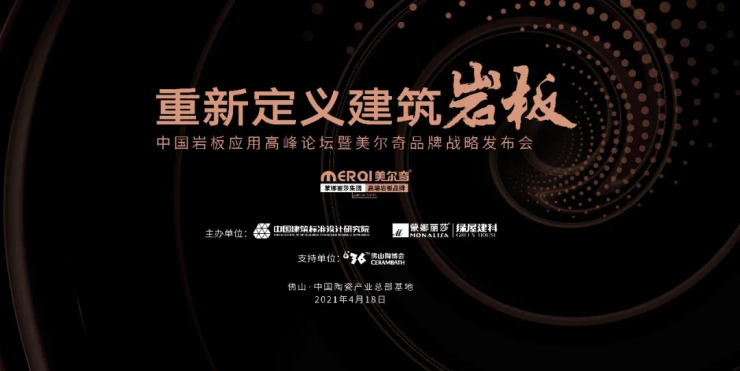8、2021中国岩板峰会

2021中国岩板峰会将于4月20日举行。本次大会由中国陶瓷总部 | 中国岩板·新材展示采购基地和陶业要闻摘要联合主办，岩板show等单位协办，以“跨域岩板 谋定未来”为主题，共同打造关注岩板趋势的思想盛宴，聚焦岩板发展的行业交流盛会。9、2021国际建筑材料创新应用交流沙龙10、新机遇，知动向—佛山陶博会海外直播逛展11、岩板定制家居分会成立筹备会议暨岩板定制家居产业研讨会

4月19日，由中国建筑卫生陶瓷协会、佛山中国陶瓷城集团、中国陶瓷总部 | 中国岩板·新材展示采购基地联合举办的岩板定制家居分会成立筹备会议暨岩板定制家居产业研讨会在中国陶瓷总部·陶瓷剧场3楼多功能会议室举办。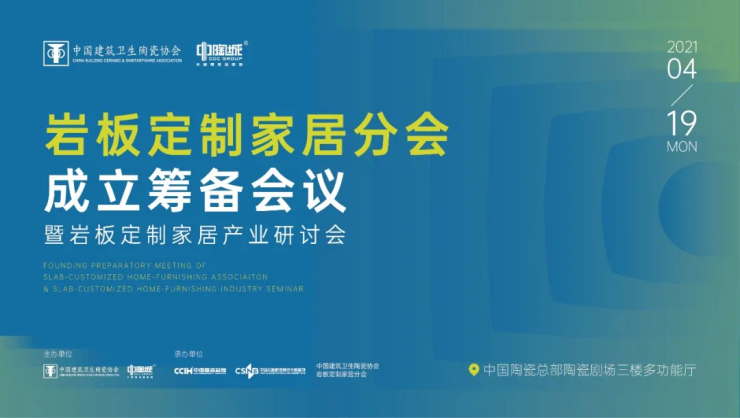......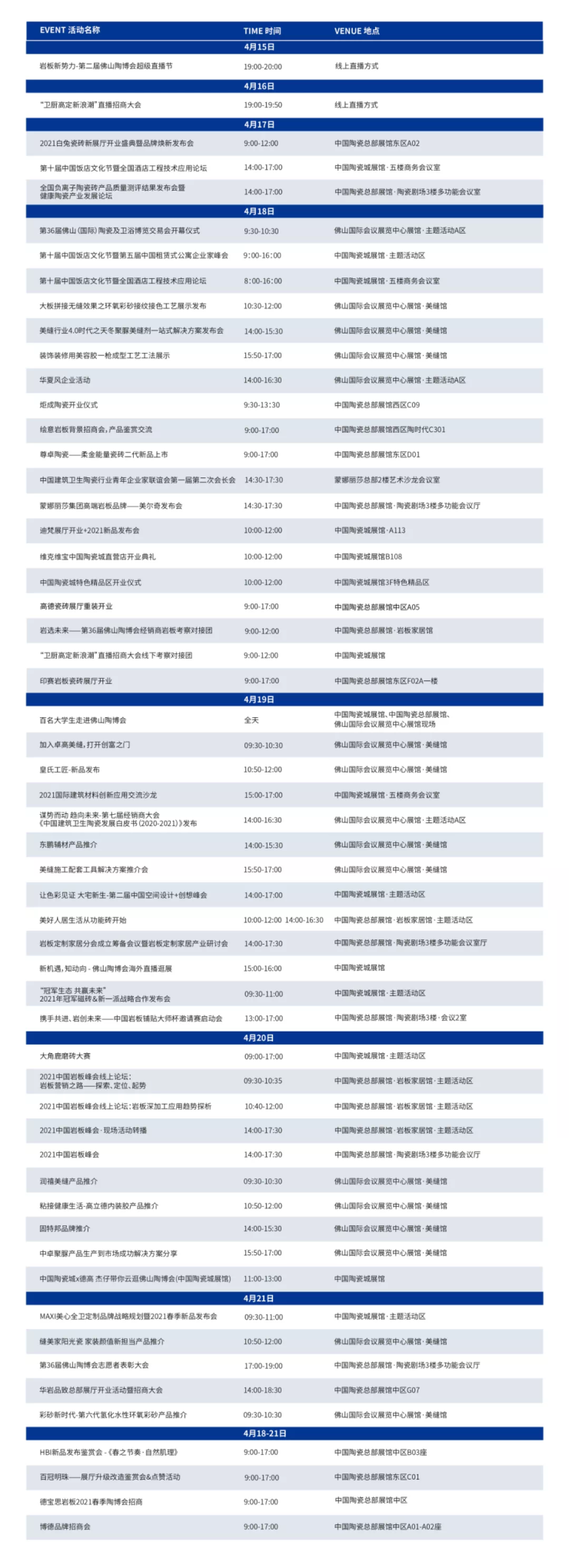▲请上下滑动查看，点击查看大图

（注：活动以实际发生为准）

Part

03

👇展会地址 👇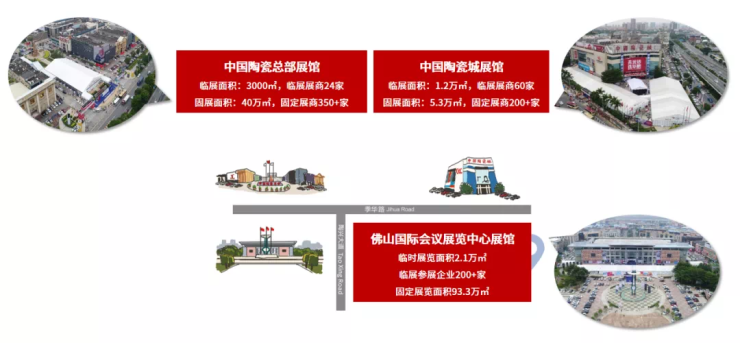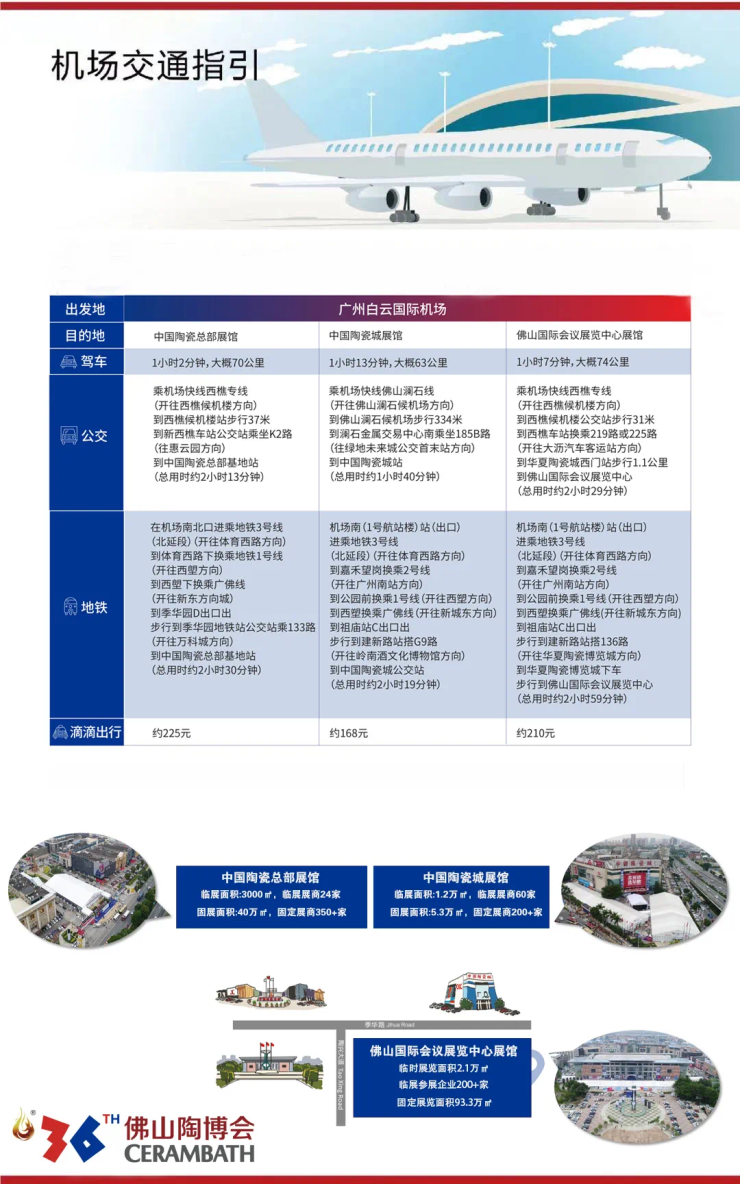▲点击查看大图

Part

04

2楼高尔思咖啡、1楼麦当劳、1楼7-11

Mof、沙县小吃、衡阳原味鱼粉、齿灵香、迎客来餐馆、江西米粉、卡索休闲饮品、7-11 便利店、宴福、宜宾燃面、H BAR、重庆三碗面、LA‘BOBO CAFE

一些柠檬一些茶、宴福

Part

05▲点击查看大图

Part

06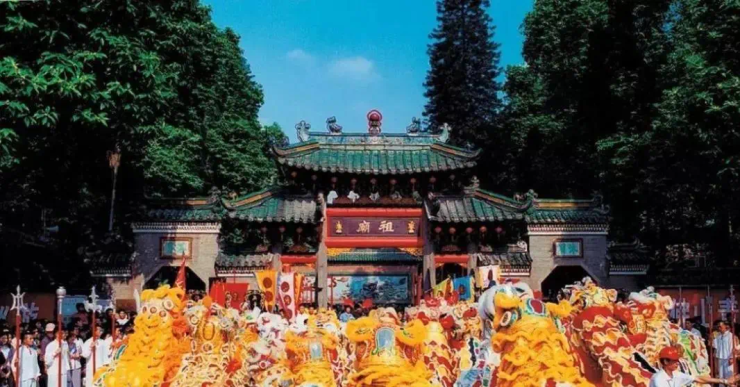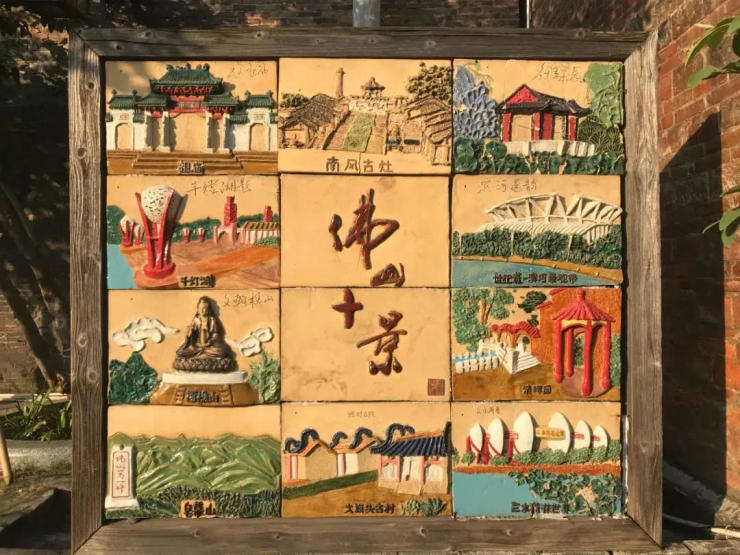▲图片来源于网络

Part

07

01 祈祷室

👉中国陶瓷城5楼B区手扶梯旁（即B501郁金香展厅旁边）

👉佛山国际会议展览中心展馆内大门右侧手扶梯附近

02 订机票/火车票/酒店/翻译/租车/租会议室/物流等服务

👉 中国陶瓷城展馆东门大堂1楼展会服务中心

0757-82729999

👉 中国陶瓷总部展馆陶瓷剧场1楼买家服务中心

0757-82526666

👉 佛山国际会议展览中心展馆买家服务中心

0757-82521999

03 银行或ATM

👉 中国陶瓷城1楼：中国工商银行、中国邮政、中国银行、 中国农业银行

👉 中国陶瓷总部西区F01：佛山农商银行

👉 佛山国际会议展览中心展馆：中国工商银行、佛山农商银行、中国银行

♦温馨提示

1、为确保人员安全快速到达展会现场，请提前关注市轨道集团发布的相关信息。

2、为缓解展会期间停车场压力，请参展商、观众尽量选择公共交通到达展馆，三展馆间穿梭选择官方免费穿梭巴士。

3、近期广东天气多变，请提前关注佛山市天气预报，带好防寒保暖衣物以及防晒霜、雨伞等个人用品。

4月18-21日

`声明：本文由入驻焦点开放平台的作者撰写，除焦点官方账号外，观点仅代表作者本人，不代表焦点立场错误信息举报电话： 400-099-0099，邮箱：jubao@vip.sohu.com，或点此进行意见反馈，或点此进行举报投诉。`

A B C D E F G H J K L M N P Q R S T W X Y Z
A - B - C - D - E
• A
• 鞍山
• 安庆
• 安阳
• 安顺
• 安康
• 澳门
• B
• 北京
• 保定
• 包头
• 巴彦淖尔
• 本溪
• 蚌埠
• 亳州
• 滨州
• 北海
• 百色
• 巴中
• 毕节
• 保山
• 宝鸡
• 白银
• 巴州
• C
• 承德
• 沧州
• 长治
• 赤峰
• 朝阳
• 长春
• 常州
• 滁州
• 池州
• 长沙
• 常德
• 郴州
• 潮州
• 崇左
• 重庆
• 成都
• 楚雄
• 昌都
• 慈溪
• 常熟
• D
• 大同
• 大连
• 丹东
• 大庆
• 东营
• 德州
• 东莞
• 德阳
• 达州
• 大理
• 德宏
• 定西
• 儋州
• 东平
• E
• 鄂尔多斯
• 鄂州
• 恩施
F - G - H - I - J
• F
• 抚顺
• 阜新
• 阜阳
• 福州
• 抚州
• 佛山
• 防城港
• G
• 赣州
• 广州
• 桂林
• 贵港
• 广元
• 广安
• 贵阳
• 固原
• H
• 邯郸
• 衡水
• 呼和浩特
• 呼伦贝尔
• 葫芦岛
• 哈尔滨
• 黑河
• 淮安
• 杭州
• 湖州
• 合肥
• 淮南
• 淮北
• 黄山
• 菏泽
• 鹤壁
• 黄石
• 黄冈
• 衡阳
• 怀化
• 惠州
• 河源
• 贺州
• 河池
• 海口
• 红河
• 汉中
• 海东
• 怀来
• I
• J
• 晋中
• 锦州
• 吉林
• 鸡西
• 佳木斯
• 嘉兴
• 金华
• 景德镇
• 九江
• 吉安
• 济南
• 济宁
• 焦作
• 荆门
• 荆州
• 江门
• 揭阳
• 金昌
• 酒泉
• 嘉峪关
K - L - M - N - P
• K
• 开封
• 昆明
• 昆山
• L
• 廊坊
• 临汾
• 辽阳
• 连云港
• 丽水
• 六安
• 龙岩
• 莱芜
• 临沂
• 聊城
• 洛阳
• 漯河
• 娄底
• 柳州
• 来宾
• 泸州
• 乐山
• 六盘水
• 丽江
• 临沧
• 拉萨
• 林芝
• 兰州
• 陇南
• M
• 牡丹江
• 马鞍山
• 茂名
• 梅州
• 绵阳
• 眉山
• N
• 南京
• 南通
• 宁波
• 南平
• 宁德
• 南昌
• 南阳
• 南宁
• 内江
• 南充
• P
• 盘锦
• 莆田
• 平顶山
• 濮阳
• 攀枝花
• 普洱
• 平凉
Q - R - S - T - W
• Q
• 秦皇岛
• 齐齐哈尔
• 衢州
• 泉州
• 青岛
• 清远
• 钦州
• 黔南
• 曲靖
• 庆阳
• R
• 日照
• 日喀则
• S
• 石家庄
• 沈阳
• 双鸭山
• 绥化
• 上海
• 苏州
• 宿迁
• 绍兴
• 宿州
• 三明
• 上饶
• 三门峡
• 商丘
• 十堰
• 随州
• 邵阳
• 韶关
• 深圳
• 汕头
• 汕尾
• 三亚
• 三沙
• 遂宁
• 山南
• 商洛
• 石嘴山
• T
• 天津
• 唐山
• 太原
• 通辽
• 铁岭
• 泰州
• 台州
• 铜陵
• 泰安
• 铜仁
• 铜川
• 天水
• 天门
• W
• 乌海
• 乌兰察布
• 无锡
• 温州
• 芜湖
• 潍坊
• 威海
• 武汉
• 梧州
• 渭南
• 武威
• 吴忠
• 乌鲁木齐
X - Y - Z
• X
• 邢台
• 徐州
• 宣城
• 厦门
• 新乡
• 许昌
• 信阳
• 襄阳
• 孝感
• 咸宁
• 湘潭
• 湘西
• 西双版纳
• 西安
• 咸阳
• 西宁
• 仙桃
• 西昌
• Y
• 运城
• 营口
• 盐城
• 扬州
• 鹰潭
• 宜春
• 烟台
• 宜昌
• 岳阳
• 益阳
• 永州
• 阳江
• 云浮
• 玉林
• 宜宾
• 雅安
• 玉溪
• 延安
• 榆林
• 银川
• Z
• 张家口
• 镇江
• 舟山
• 漳州
• 淄博
• 枣庄
• 郑州
• 周口
• 驻马店
• 株洲
• 张家界
• 珠海
• 湛江
• 肇庆
• 中山
• 自贡
• 资阳
• 遵义
• 昭通
• 张掖
• 中卫

1室1厅1厨1卫1阳台

1
2
3
4
5

0
1
2

1

1

0
1
2
3报名成功，资料已提交审核A B C D E F G H J K L M N P Q R S T W X Y Z
A - B - C - D - E
• A
• 鞍山
• 安庆
• 安阳
• 安顺
• 安康
• 澳门
• B
• 北京
• 保定
• 包头
• 巴彦淖尔
• 本溪
• 蚌埠
• 亳州
• 滨州
• 北海
• 百色
• 巴中
• 毕节
• 保山
• 宝鸡
• 白银
• 巴州
• C
• 承德
• 沧州
• 长治
• 赤峰
• 朝阳
• 长春
• 常州
• 滁州
• 池州
• 长沙
• 常德
• 郴州
• 潮州
• 崇左
• 重庆
• 成都
• 楚雄
• 昌都
• 慈溪
• 常熟
• D
• 大同
• 大连
• 丹东
• 大庆
• 东营
• 德州
• 东莞
• 德阳
• 达州
• 大理
• 德宏
• 定西
• 儋州
• 东平
• E
• 鄂尔多斯
• 鄂州
• 恩施
F - G - H - I - J
• F
• 抚顺
• 阜新
• 阜阳
• 福州
• 抚州
• 佛山
• 防城港
• G
• 赣州
• 广州
• 桂林
• 贵港
• 广元
• 广安
• 贵阳
• 固原
• H
• 邯郸
• 衡水
• 呼和浩特
• 呼伦贝尔
• 葫芦岛
• 哈尔滨
• 黑河
• 淮安
• 杭州
• 湖州
• 合肥
• 淮南
• 淮北
• 黄山
• 菏泽
• 鹤壁
• 黄石
• 黄冈
• 衡阳
• 怀化
• 惠州
• 河源
• 贺州
• 河池
• 海口
• 红河
• 汉中
• 海东
• 怀来
• I
• J
• 晋中
• 锦州
• 吉林
• 鸡西
• 佳木斯
• 嘉兴
• 金华
• 景德镇
• 九江
• 吉安
• 济南
• 济宁
• 焦作
• 荆门
• 荆州
• 江门
• 揭阳
• 金昌
• 酒泉
• 嘉峪关
K - L - M - N - P
• K
• 开封
• 昆明
• 昆山
• L
• 廊坊
• 临汾
• 辽阳
• 连云港
• 丽水
• 六安
• 龙岩
• 莱芜
• 临沂
• 聊城
• 洛阳
• 漯河
• 娄底
• 柳州
• 来宾
• 泸州
• 乐山
• 六盘水
• 丽江
• 临沧
• 拉萨
• 林芝
• 兰州
• 陇南
• M
• 牡丹江
• 马鞍山
• 茂名
• 梅州
• 绵阳
• 眉山
• N
• 南京
• 南通
• 宁波
• 南平
• 宁德
• 南昌
• 南阳
• 南宁
• 内江
• 南充
• P
• 盘锦
• 莆田
• 平顶山
• 濮阳
• 攀枝花
• 普洱
• 平凉
Q - R - S - T - W
• Q
• 秦皇岛
• 齐齐哈尔
• 衢州
• 泉州
• 青岛
• 清远
• 钦州
• 黔南
• 曲靖
• 庆阳
• R
• 日照
• 日喀则
• S
• 石家庄
• 沈阳
• 双鸭山
• 绥化
• 上海
• 苏州
• 宿迁
• 绍兴
• 宿州
• 三明
• 上饶
• 三门峡
• 商丘
• 十堰
• 随州
• 邵阳
• 韶关
• 深圳
• 汕头
• 汕尾
• 三亚
• 三沙
• 遂宁
• 山南
• 商洛
• 石嘴山
• T
• 天津
• 唐山
• 太原
• 通辽
• 铁岭
• 泰州
• 台州
• 铜陵
• 泰安
• 铜仁
• 铜川
• 天水
• 天门
• W
• 乌海
• 乌兰察布
• 无锡
• 温州
• 芜湖
• 潍坊
• 威海
• 武汉
• 梧州
• 渭南
• 武威
• 吴忠
• 乌鲁木齐
X - Y - Z
• X
• 邢台
• 徐州
• 宣城
• 厦门
• 新乡
• 许昌
• 信阳
• 襄阳
• 孝感
• 咸宁
• 湘潭
• 湘西
• 西双版纳
• 西安
• 咸阳
• 西宁
• 仙桃
• 西昌
• Y
• 运城
• 营口
• 盐城
• 扬州
• 鹰潭
• 宜春
• 烟台
• 宜昌
• 岳阳
• 益阳
• 永州
• 阳江
• 云浮
• 玉林
• 宜宾
• 雅安
• 玉溪
• 延安
• 榆林
• 银川
• Z
• 张家口
• 镇江
• 舟山
• 漳州
• 淄博
• 枣庄
• 郑州
• 周口
• 驻马店
• 株洲
• 张家界
• 珠海
• 湛江
• 肇庆
• 中山
• 自贡
• 资阳
• 遵义
• 昭通
• 张掖
• 中卫• 手机• 分享
• 设计
免费设计
• 计算器
装修计算器
• 联系
• 置顶
返回顶部